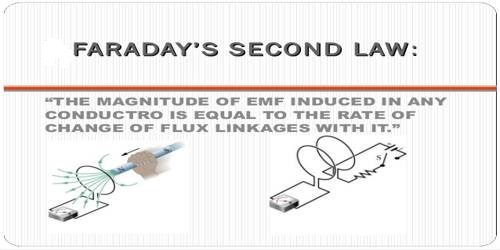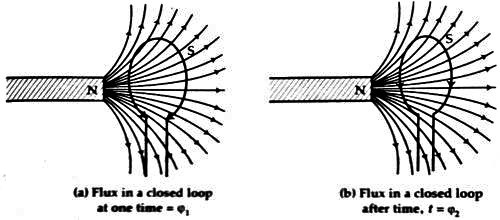# Faraday’s Second Law of Electro-magnetic Induction

Faraday’s Second Law of Electro-magnetic Induction

In 1831 famous scientist Faraday gave two laws of electro-magnetic induction. According to his name, these laws are called Faraday’s laws of electro-magnetic induction. An important industrial application of Faraday’s law is electroplating, where a material is coated with a thin metallic layer to prevent corrosion or abrasion.

Second law: The value of the Induced electromotive force in the coil is directly proportional to the rate of change of the number of magnetic field lines or magnetic flux.

Explanation: Faraday’s second law states that the mass of two substances that are liberated from electrodes, given the same applied electrical charge, is proportional to the molecular weights of the two substances.

Suppose at any moment the number of field lines or magnetic flux in a closed loop = φ1. Let the number of closed field lines at later time t be φ2 (figure).If the induced electromotive force is E, then from second law, we get,

E ∞ (φ2 – φ1) / t

If, (φ2 – φ1) = φ, then

E ∞ φ / t

or, E = K (φ / t) …. …. (1)

Here K is a proportionality constant whose value depends on the unit measurement. If φ is expressed in Weber, ‘t’ in second and induced electromotive force in volt, i.e., if physical quantities are expressed in S. I. unit, then K = 1.

So, from equation (1) we get, E = φ / t)

This law can be expressed by calculus. If in time dt, the change of flux is dφ, then

E = dφ/dt

If there are N number of turns in the coil, then,

E = N (dφ/dt)

Newmann gave the mathematical form of the second law of Faraday. So it is known as Newmann’s law.